Courses

# Network Synthesis - 1

## 10 Questions MCQ Test Topicwise Question Bank for Electronics Engineering | Network Synthesis - 1

Description
This mock test of Network Synthesis - 1 for Electrical Engineering (EE) helps you for every Electrical Engineering (EE) entrance exam. This contains 10 Multiple Choice Questions for Electrical Engineering (EE) Network Synthesis - 1 (mcq) to study with solutions a complete question bank. The solved questions answers in this Network Synthesis - 1 quiz give you a good mix of easy questions and tough questions. Electrical Engineering (EE) students definitely take this Network Synthesis - 1 exercise for a better result in the exam. You can find other Network Synthesis - 1 extra questions, long questions & short questions for Electrical Engineering (EE) on EduRev as well by searching above.
QUESTION: 1

### Which of the following is a positive real function?

Solution: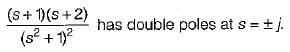Hence, notaPRF.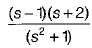has a zero (s = 1) in RH s-plane.

Hence, not a PRF.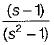has a pole and a zero in RH s-piane.
Hence, not a PRF.
Option (b) is a PRF as all poles and zeros lie in LH s-plane.

QUESTION: 2

Solution:
QUESTION: 3

### Which of the following property does not relates to LC impedance or admittance functions?

Solution:
QUESTION: 4

If the impulse response is realisable by delaying it appropriately and is bounded for bounded excitation, then the system is said to be

Solution:

Since system is bounded for bounded excitation therefore, it will be stable.
Since the system is delayed therefore, output may exist before the input is applied.
Hence, the system will be non-causal.

QUESTION: 5

A network-A has zeros only in the left-half of the s-plane while the network-B has zeros only in the right-half of the s-plane. The network-A and Network-B will be respectively called

Solution:
QUESTION: 6

An L-C admittance or impedance function

Solution:
QUESTION: 7

If F1(s) and F2(s) are positive real functions, then which of the following are positive real function?

Solution:
QUESTION: 8

For the pole-zero plot shown below, the network function is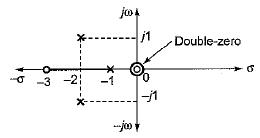Solution:

Here, zeros are at: s = 0, 0,-3

Poles are at s = - 1,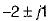or, poles are at (s + 2)2 + 1 - 0 and at s = -1 or, s2 + 4s + 5 = 0 and at s = -1

QUESTION: 9

A stable system must have

Solution:
QUESTION: 10

Which of the following is not a positive real function?

Solution: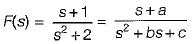Here, a = 1 , b = 0, c = 2

Thus, a, b, c ≥ 0 but b < a

For PRF, b ≥ a therefore, option (d) is not a PRF.